## Riya and Maya are two friends studying in the same class. While doing their mathematics project on Relation and  Functions they have to collect the name of five metro cities and four cities other than metro cities of India; and  present the name of cities in the form of sets. They have collected the names of cities and write the in the form of  sets given as follows:  A = {Five Metro cities of India} = {Delhi, Mumbai, Bangalore, Calcutta, Pune}  B = {Bihar, Agra, Jaipur, Ahmedabad}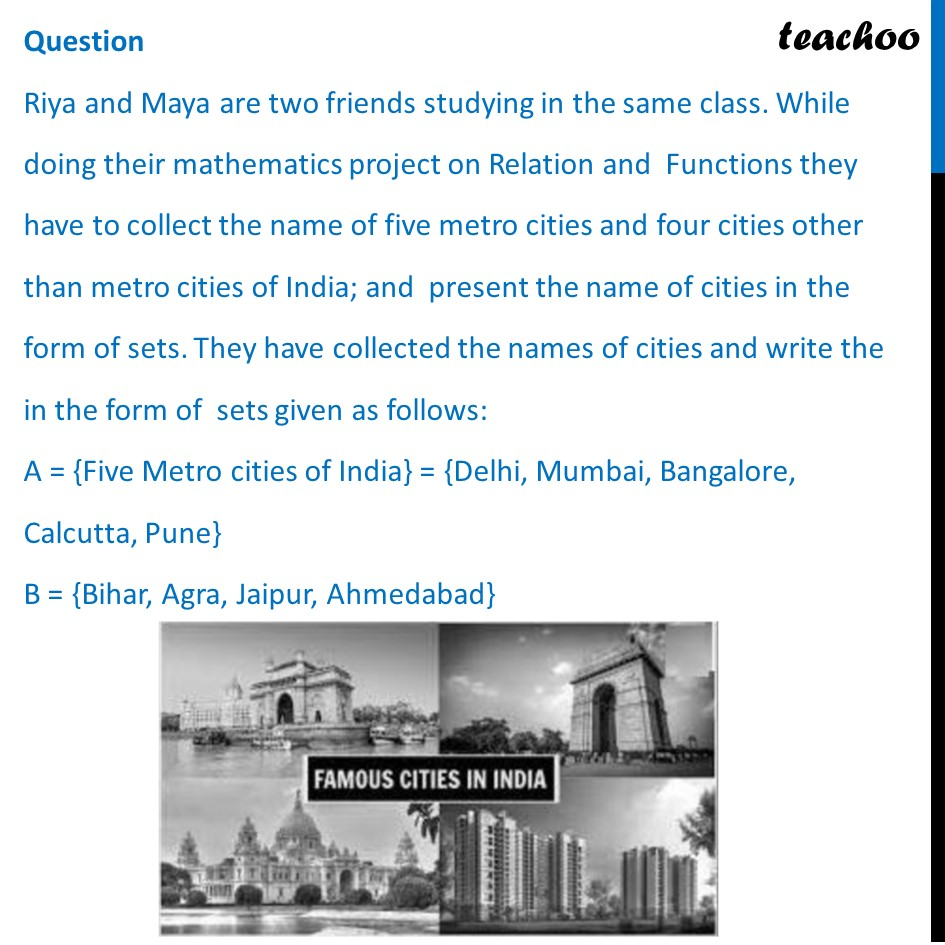## How many functions exist from Set A to Set B?  (a) 512     (b) 1024  (c) 2048   (d) 254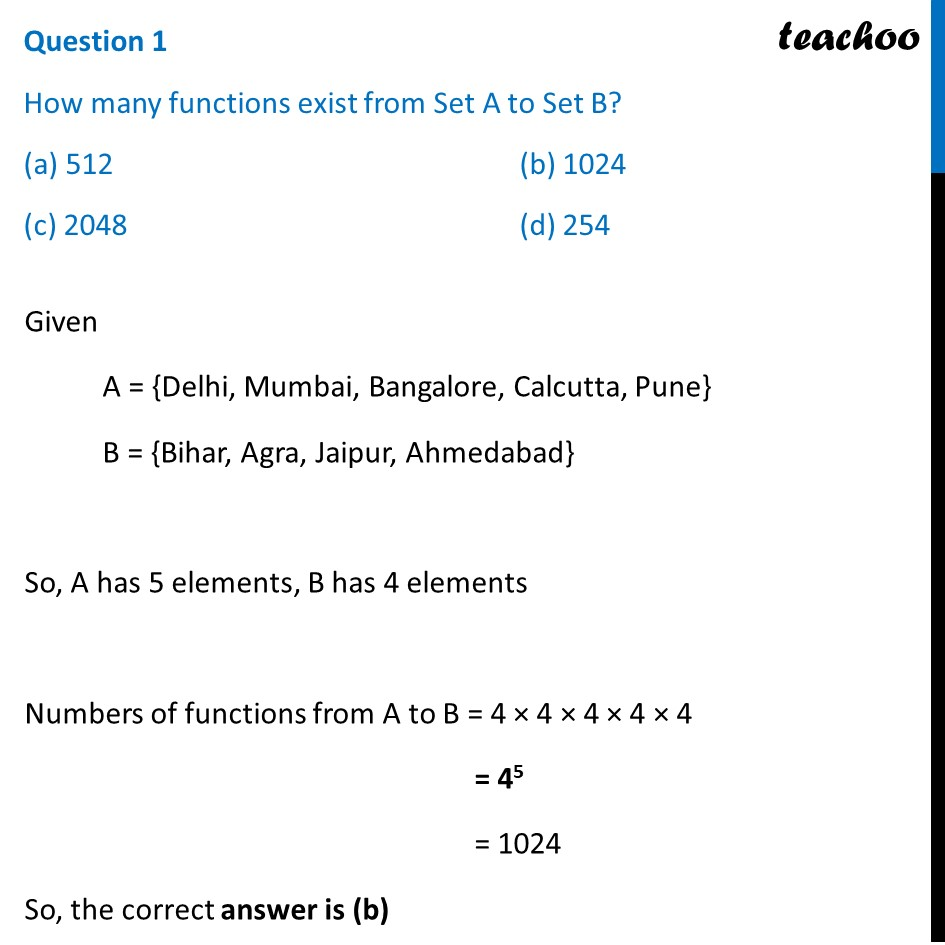## Riya wants to know how many relations are possible from set A to set B?  (a) 220   (b) 210  (c) 225   (d) 512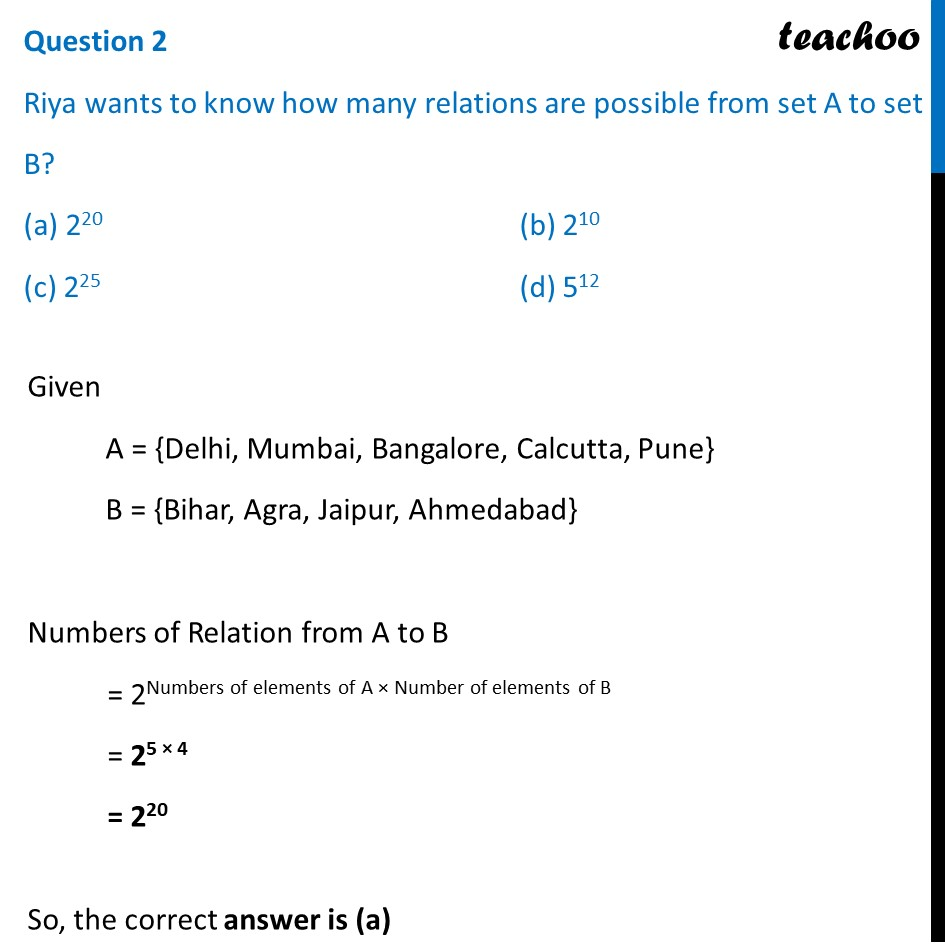## (a) Reflexive   (b) Symmetric  (c) Both Reflexive and Symmetric (d) Neither Reflexive nor Symmetric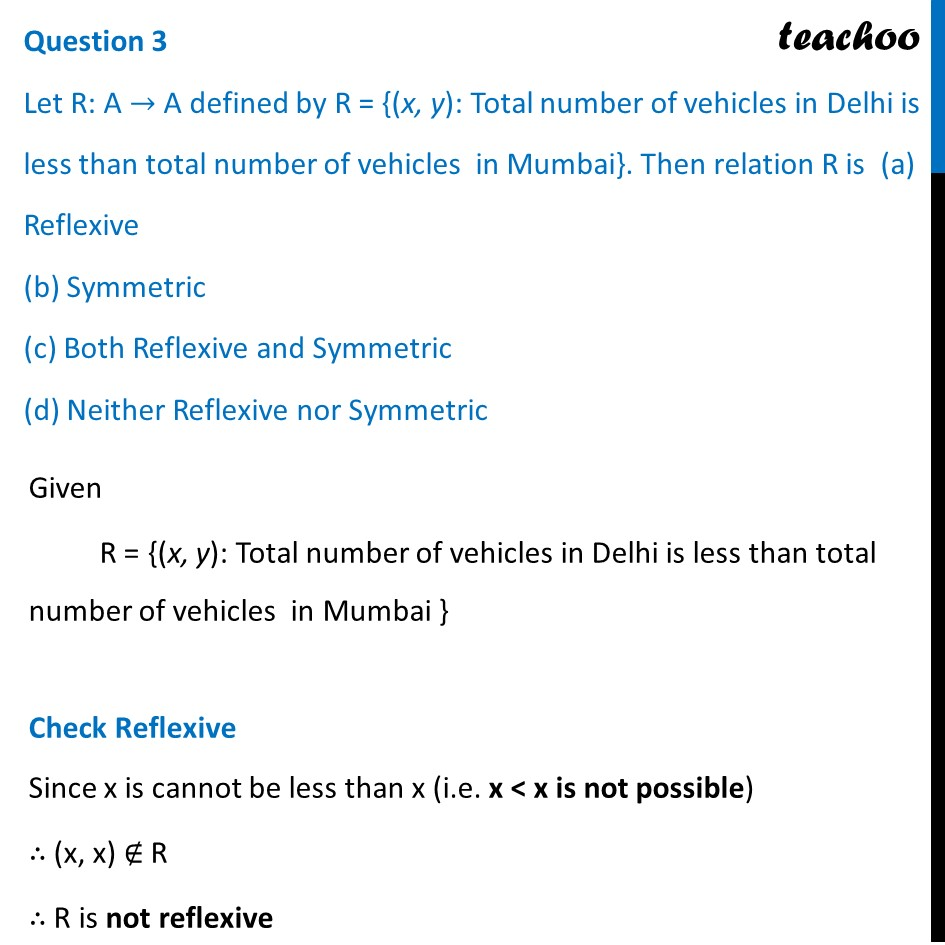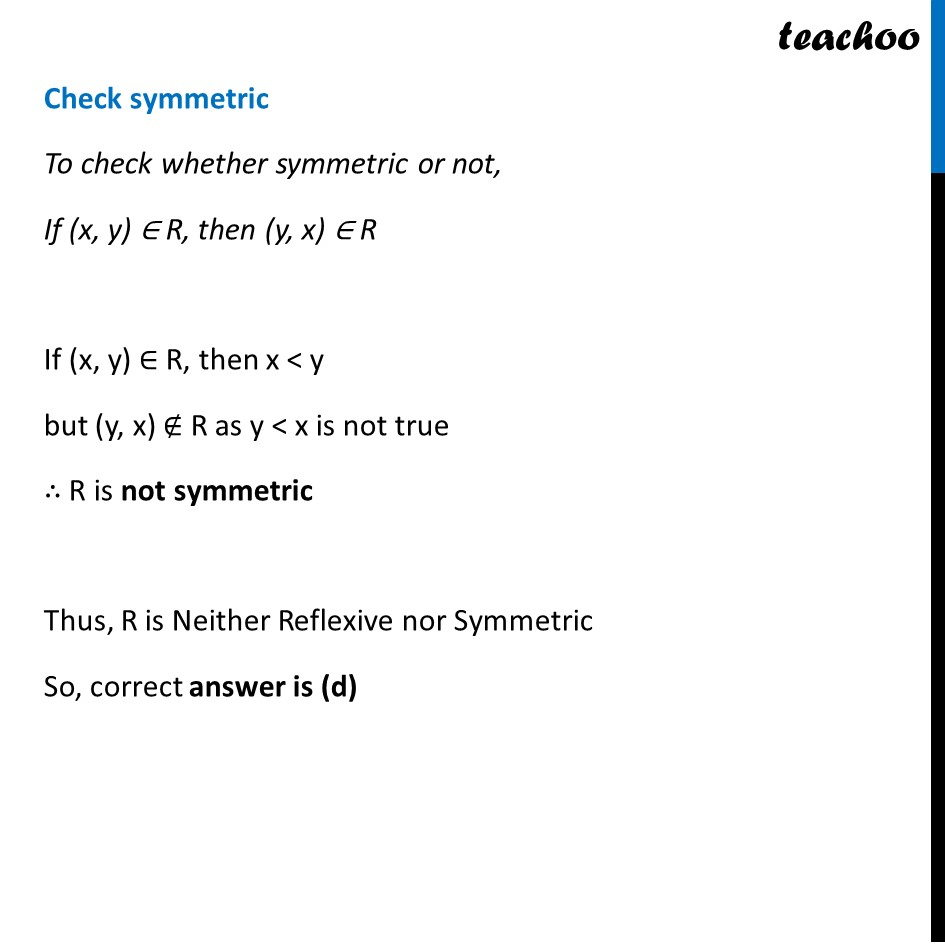## How many reflexive relations can be defined on set B?  (a) 25   (b) 210   (c) 412   (d) 212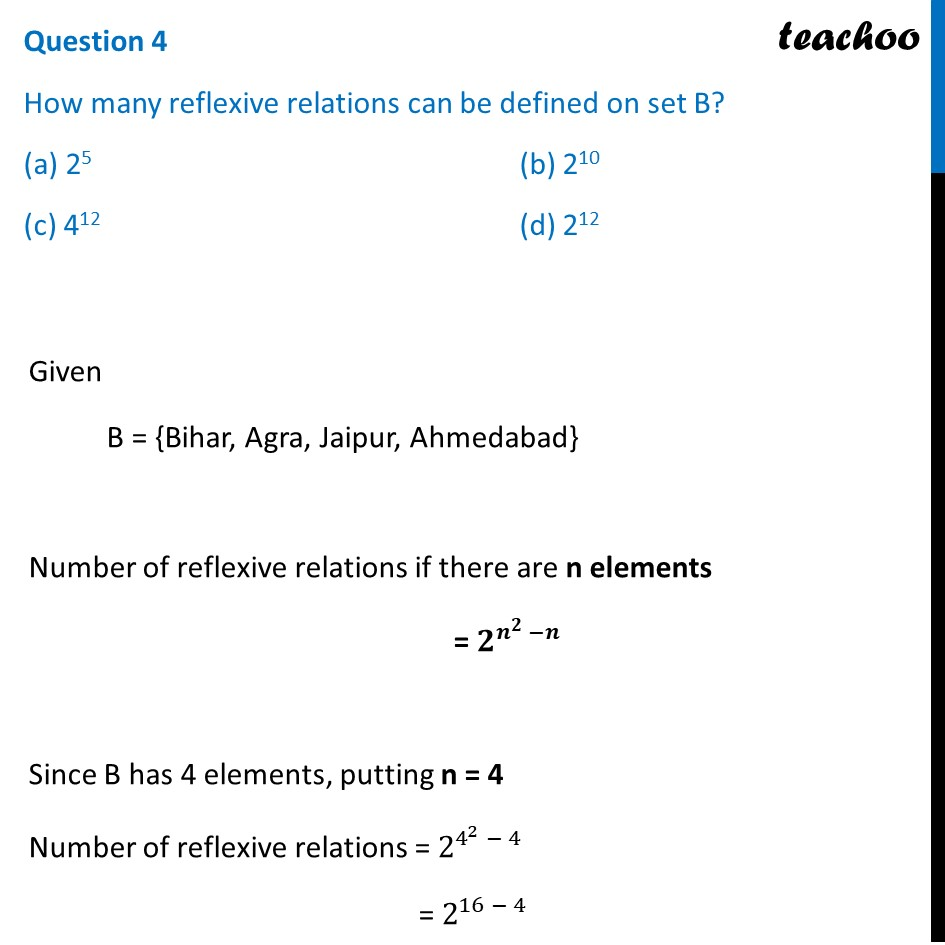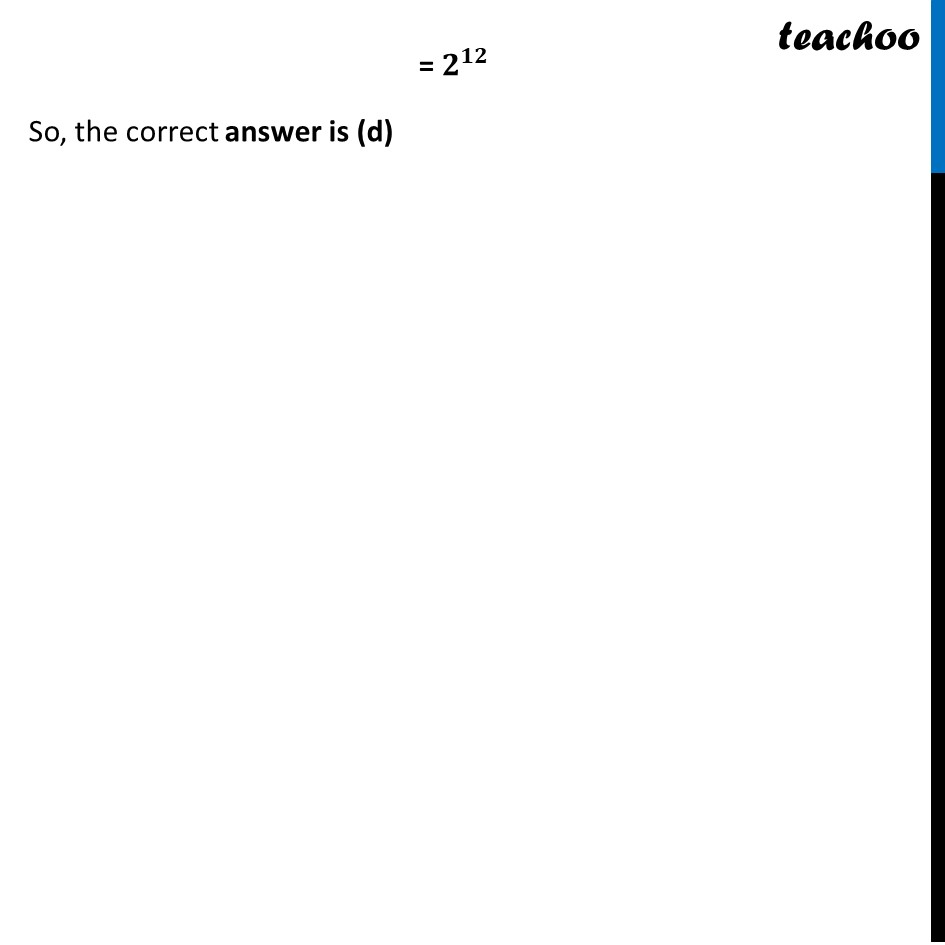## How many symmetric relations defined on set A? (a) 25   (b) 210   (c) 215   (d) 26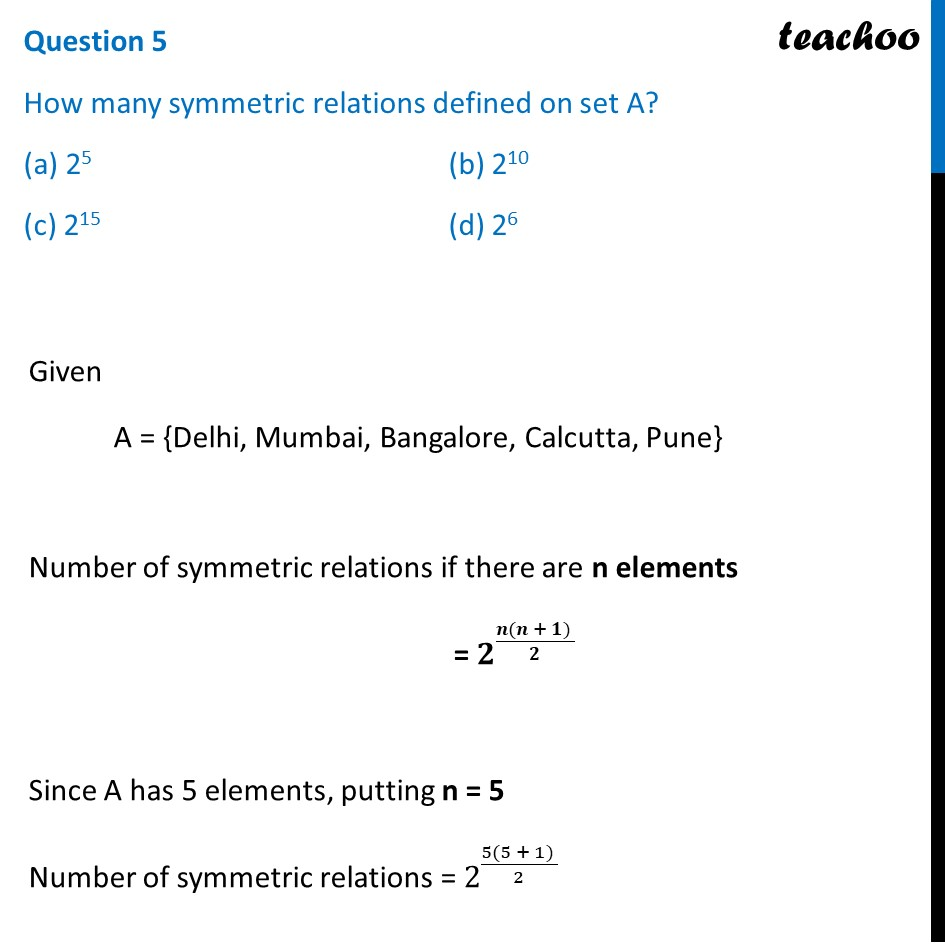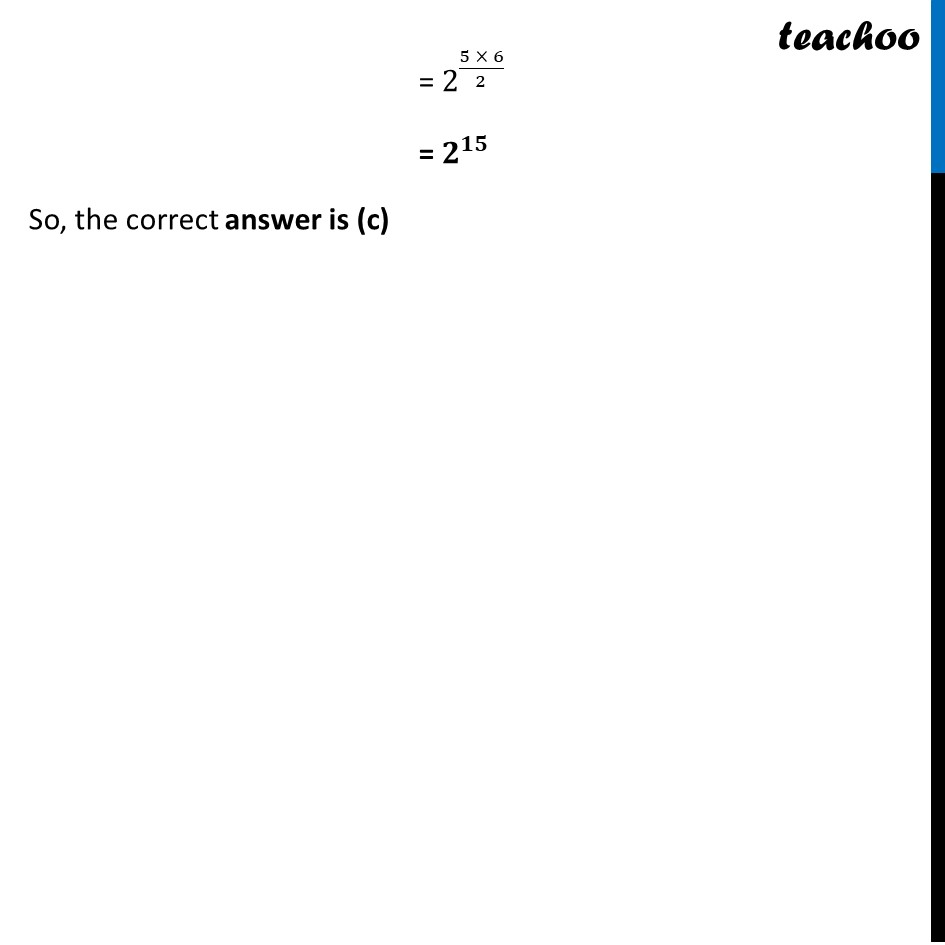1. Chapter 1 Class 12 Relation and Functions (Term 1)
2. Serial order wise
3. Case Based Questions (MCQ)

Transcript

Question Riya and Maya are two friends studying in the same class. While doing their mathematics project on Relation and Functions they have to collect the name of five metro cities and four cities other than metro cities of India; and present the name of cities in the form of sets. They have collected the names of cities and write the in the form of sets given as follows: A = {Five Metro cities of India} = {Delhi, Mumbai, Bangalore, Calcutta, Pune} B = {Bihar, Agra, Jaipur, Ahmedabad} Question 1 How many functions exist from Set A to Set B? (a) 512 (b) 1024 (c) 2048 (d) 254 Given A = {Delhi, Mumbai, Bangalore, Calcutta, Pune} B = {Bihar, Agra, Jaipur, Ahmedabad} So, A has 5 elements, B has 4 elements Numbers of functions from A to B = 4 × 4 × 4 × 4 × 4 = 45 = 1024 So, the correct answer is (b) Question 2 Riya wants to know how many relations are possible from set A to set B? (a) 220 (b) 210 (c) 225 (d) 512 Given A = {Delhi, Mumbai, Bangalore, Calcutta, Pune} B = {Bihar, Agra, Jaipur, Ahmedabad} Numbers of Relation from A to B = 2Numbers of elements of A × Number of elements of B = 25 × 4 = 220 So, the correct answer is (a) Question 3 Let R: A → A defined by R = {(x, y): Total number of vehicles in Delhi is less than total number of vehicles in Mumbai}. Then relation R is (a) Reflexive (b) Symmetric (c) Both Reflexive and Symmetric (d) Neither Reflexive nor Symmetric Given R = {(x, y): Total number of vehicles in Delhi is less than total number of vehicles in Mumbai } Check Reflexive Since x is cannot be less than x (i.e. x < x is not possible) ∴ (x, x) ∉ R ∴ R is not reflexive Check symmetric To check whether symmetric or not, If (x, y) ∈ R, then (y, x) ∈ R If (x, y) ∈ R, then x < y but (y, x) ∉ R as y < x is not true ∴ R is not symmetric Thus, R is Neither Reflexive nor Symmetric So, correct answer is (d) Question 4 How many reflexive relations can be defined on set B? (a) 25 (b) 210 (c) 412 (d) 212 Given B = {Bihar, Agra, Jaipur, Ahmedabad} Number of reflexive relations if there are n elements = 𝟐^(𝒏^𝟐 −𝒏) Since B has 4 elements, putting n = 4 Number of reflexive relations = 2^(4^2 − 4) = 2^(16 − 4) = 𝟐^𝟏𝟐 So, the correct answer is (d) Question 5 How many symmetric relations defined on set A? (a) 25 (b) 210 (c) 215 (d) 26 Given A = {Delhi, Mumbai, Bangalore, Calcutta, Pune} Number of symmetric relations if there are n elements = 𝟐^((𝒏(𝒏 + 𝟏) )/𝟐 ) Since A has 5 elements, putting n = 5 Number of symmetric relations = 2^((5(5 + 1) )/2) = 2^((5 × 6)/2) = 𝟐^𝟏𝟓 So, the correct answer is (c)